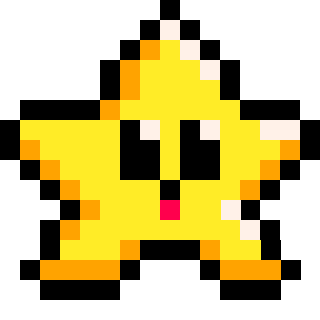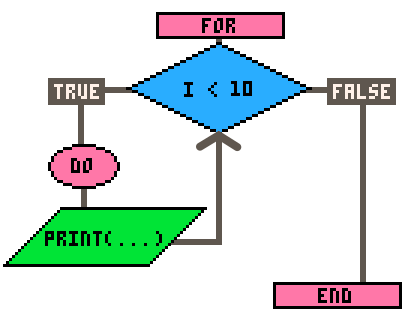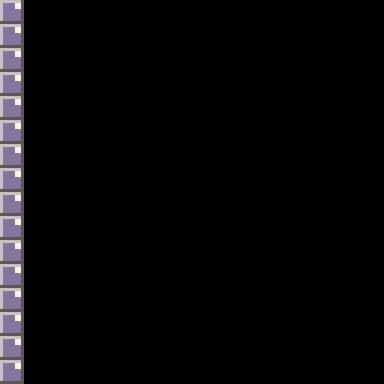# loops

This loop will repeat the code after `do` for each entry of a numbered table provided and it will also set the value for each table entry as the local variable names you give them after `for`.

``for variable in all( table ) do``
 `value` a local variable name (used within this loop) that will hold the value data (right column data of the table) `table` variable name of a numbered table

Example Table:

 HighScores john 143 bob 99 kim 984
``````for score in all( highscores )
print( score )
end

-- prints: 10 143 984``````105

18 Mar 2023

This loop will repeat the function for each entry of a numbered table as if you call the function and pass the value of the table to the function.

``foreach( table, function )``
 `table` variable name of a numbered table `function` a function name, without parentheses, that will be called to run repeatedly and given the value of each table entry each time it is run.

Example Table:

 HighScores john 143 bob 99 kim 984
``````foreach( highscores, print )

-- prints: 10 143 984``````93

18 Mar 2023

This loop will repeat the code after `do` for each entry of the table provided and it will also set both the key and the value for each table entry as the local variable names you give them after `for`.

``for key, value in pairs( table ) do``
 `key` a local variable name (used within this loop) that will hold the key name (left column data) `value` a local variable name (used within this loop) that will hold the value data (right column data of the table) `table` variable name of a numbered table

Example Table:

 HighScores john 143 bob 99 kim 984

This is how you loop through this table, being able to access and use both the keys (names) and values (scores):

``````for name, score in pairs( highscores )
print( name )
print( score )
end

-- prints: john 10 bob 143 kim 984``````76

18 Mar 2023

``````for variable=start, stop, [count_by] do
--code to repeat
end``````

 `variable` Often named as `i` to mean the "index" number of the loop. This number will indicate the current count of the loop. `start` A number, often set to `0` or `1` depending on how you might use the variable to keep track of the loop count and current index number. `stop` A number, used to end the loop when the index variable reaches beyond this stop number. `count by` (optional, default 1) A number, added to the index variable before the loop repeats. Negative numbers will decreas the index number.

"For loops" begin counting from the "start" number, count up or down by the "count by" number, and stops after reaching the "stop" number.

A "for loop" is a programming structure that allows you to repeat a set of instructions multiple times. It's like a machine that can do the same task over and over again. You tell the machines how many times to repeat the actions it is meant to do, and it does the rest. It's very useful for doing repetitive tasks quickly and efficiently.###### Examples:

``````for i=1, 9, 2 do
print( i )
end

--prints: 1 3 5 7 9``````

If the "count by" number is left out, then it will automatically be 1.

``````for i=1, 9 do
print( i )
end

--prints: 1 2 3 4 5 6 7 8 9``````

If you want to count down, then make the "count by" number a negative number.

``````for i=10, 3, -1 do
print( i )
end

--prints: 10 9 8 7 6 5 4 3``````

It's important to note that the index number is not checked until the end of each loop, so even when the "start" and "stop" numbers are the same, the loop will still run once through.

``````for i=0, 0 do
print( i )
end

--prints: 0``````

You may come across or need to use a double loop, or a loop within a loop. These are called "nested loops". Here is an example:

``````for i=0, 3 do
for j=1, 2 do
print( j )
end
print( "i="..i )
end

--prints: 1 2 i=0 1 2 i=1 1 2 i=2 1 2 i=3``````

You can differentiate the two loops by saying "outer" and "inner" loop. Just as it is common to use `i` as the index variable name, it is also common to then use the next letters, `j`, `k`, etc. for each inner loop. Notice that the index number doesn't change109

17 Mar 2023

``````while condition do
--code to repeat
end``````
 `condition` a variable or statement that will resolve to true or false. The code inside of this loop will repeat for as long as this condition is true.

"WHILE LOOPS" repeat the code after DO and stops repeating when the condition is false.

Example:

``````b = 4
while b < 10 do
print( b )
b += 1
end

--prints: 4 5 6 7 8 9``````83

18 Mar 2023

``break``

Example:

``````for i = 0, 10 do
if i == 5 then
break
end
print ( i )
end

--prints 0 1 2 3 4
``````71

18 Mar 2023

A loop is a feature of programming that allows you to repeat a specific block of code multiple times until receiving the order to stop. They are useful when you need to perform a task multiple times, or when you need to iterate (go over) a set of data. Most loops will repeat until a certain condition is met.

The most common loop is the `for` loop.

``````for i=0, 10, 1 do
print(i)
end

--prints 0 1 2 3 4 5 6 7 8 9 10``````

Here is a flowchart to visualize this code:This loop keeps track of a variable (most use the letter `i` like this), which we set to start at `0`, and at the end of each repitition it increases by the third number, `1`. Before starting the next repitition, it checks the condition and compares `i` with the second number given, `10`. If `i` is less than `10`, then the condition is true and the loop continues. Only when the condition is false, does the loop end.

There are different types of loops that are unique in how they handle what they repeat and when they stop, so it is important to learn them so you can choose the best type of loop for what you need.#### Types of Loops

For - used to execute a block of code a fixed number of times, typically by iterating over a range of values.

While - used to execute a block of code repeatedly as long as a certain condition is true.

Foreach - used to iterate over the entries of a table and pass each entry to a function.

For in all - used to iterate over all entries of a numeric table and set each entry as a local variable.

For in pairs - used to iterate over the key-value pairs of a table.

Repeat Until - similar to a while loop, but it executes the block of code at least once, and then repeats it until a certain condition is true.#### Common Uses of Loops

Loops are often used with sets of data (tables) to update, compare, or draw each entry in the set. Loops are helpful to simplify complex code. This is useful in:

Physics - To apply forces such as gravity and friction to multiple objects' movement variables, you can use a loop.

``foreach( object, apply_gravity )``

Animation - To cycle through sprites for multiple objects, you can use a loop.

``for f in all(flowers) do f.sprite+=1 end``

Particle systems - To change the position, size, or color of each particle in a table, you can use a loop.

``foreach( particle, update_particles )``

Pathfinding - To compare multiple enemy with tiles on the map to determine the fastest route, you can use a loop inside another loop.

``````for e in all(enemies) do
for t in all(tiles) do
--compare each enemy data with each tile data
end
end``````

Collision Detection - To check collision of a player with a list of bullets, you can use a loop.

``````for key,bullet in pairs(bullets) do
if player.x==bullet.x and player.y==bullet.y then
--player hit
end
end``````

Procedural Generation - To build random levels and maps using only code, you can use a couple loops.

``````for column=1, 16 do
for row=1, 16 do
--draw certain background tile
end
end``````#### Code Efficiency with Loops

Whenever you are copy/pasting code, consider how you could use a loop instead.

For example, we could draw wall tiles on the left side of the screen like this:``````local x=0
local y=0
local tile_size=8

spr(1, x, y + tile_size * 0)
spr(1, x, y + tile_size * 1)
spr(1, x, y + tile_size * 2)
spr(1, x, y + tile_size * 3)
spr(1, x, y + tile_size * 4)
spr(1, x, y + tile_size * 5)
spr(1, x, y + tile_size * 6)
spr(1, x, y + tile_size * 7)
spr(1, x, y + tile_size * 8)
spr(1, x, y + tile_size * 9)
spr(1, x, y + tile_size * 10)
spr(1, x, y + tile_size * 11)
spr(1, x, y + tile_size * 12)
spr(1, x, y + tile_size * 13)
spr(1, x, y + tile_size * 14)
spr(1, x, y + tile_size * 15)

--20 lines, 153 tokens``````

Or, we could simply use a loop to do the exact same thing, like this:``````local tile_size = 8
local x = 0
local screen_height = 128

for row = 0, screen_height/tile_size-1 do
local y = row * tile_size
spr(1, x, y)
end

--8 lines, 31 tokens``````

Notice how the longer code was copy pasted many times, and the only thing that changed in each line was the number multiplied at the end. The for loop counts up automatically, just like the number we want to multiply. So we can use a for loop and set the interation number to a variable named `row` and simply multiply each row by the tile size to make the y position one more tile lower each time the loop repeats.

Not only are the number of lines 60% less, but the number of tokens used in PICO-8 is 80% less. In almost all cases, using loops will greatly reduce the amount of code you write and tokens you use.

If you are just starting out and learning to use loops. Start by writing out the long code by copy and pasting many lines yourself to get it working the way you want it. Then practice trying to convert that code into a loop. That is a great way to learn because eventually, you will be able to foresee when you should use a loop, and figure out how to use it before writing it all out first.

Writing inefficient code, then making it more efficient is a never-ending practice among programmers, so don't get stuck worrying if your first draft is the most efficient it can be. Just get it working first, then make it more efficient later.#### Beware of Infinite Loops

If not handled properly, a loop can cause the computer or a program to become slower or even crash as it becomes overwhelmed with repeating the same steps in an endless loop. Some causes for infinite loop crashes are:
(1) the condition to stop the loop is never met;
(2) your loop may not have any break points;
(3) the process is too long and complicated;
(4) the data set being looped through is too large.58

19 Apr 2023

`Font`